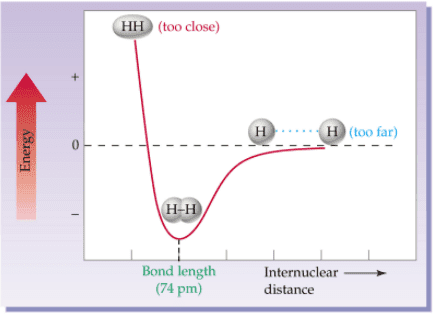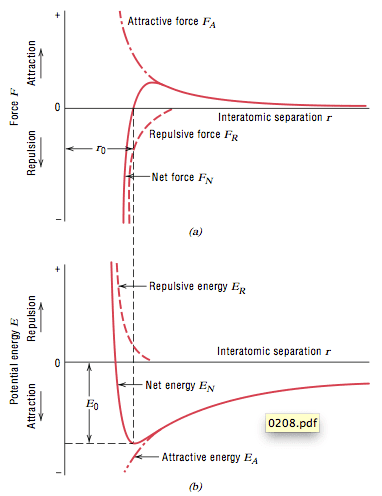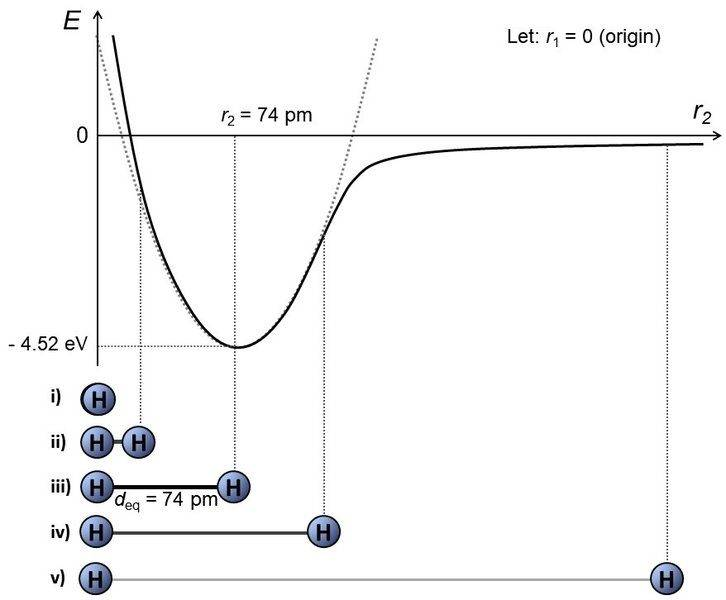# Potential energy and internuclear distance

• Daneil Leite

#### Daneil LeiteWhat exactly is this potential energy? I was searching and its looks like electric potential energy (U = K*Q*q/d - K*Q*q /d0), because the two atoms attract each other that means that the product of Q*q = negative, so, a negative energy. Ok, that's make sense, but why when the repulsive forces and attractive forces enter in equilibrium the energy still negative ? Does not that means the molecules keep attracting and coming closer and closer ? I already searched about it, but I can't understand this part.

#### Attachments

why when the repulsive forces and attractive forces enter in equilibrium the energy still negative ? Does not that means the molecules keep attracting and coming closer and closer ?
You seem to be confusing energy with force. Conservative force like the one relevant in this case is equal to the gradient of potential energy. In the minimum of a potential energy curve, the gradient is zero and thus the net force is zero - the particles are stable. So, no, the molecules will not get closer and closer as it reaches equilibrium.
because the two atoms attract each other that means that the product of Q*q = negative
It's not quite right to assign charges for the molecules in question because in fact that potential energy curve is not obtained using the usual electrostatic potential energy formula. It is obtained by solving Schroedinger equation for the electronic part of the total wavefunction of the two H atoms. It just happens that the ground state energy of H2 molecule so obtained takes a shape that resembles electrostatic potential energy.

You seem to be confusing energy with force.
Yeah, I searched for graphs of force and energy and I found this:Ok, so the forces are at equilibrium at minimum energy stage, but I still don't understand what this negative energy means. I found and animation who says that the energy is negative because the electrical potential energy turns into kinect energy, and the zero at infinite distance is only a referencial. But still, it does not make sense to me. What means to have, for example, -3 J of negative potential energy ?

#### Attachments

Ok, so the forces are at equilibrium at minimum energy stage, but I still don't understand what this negative energy means.
The absolute value of the potential energy does not matter, what governs the dynamical behavior of a system is the gradient of the potential energy which is proportional to a force. For instance, if you shifted those curves up or down, the gradient at each point remains unchanged because the derivative of a constant is zero.

I found and animation who says that the energy is negative because the electrical potential energy turns into kinect energy, and the zero at infinite distance is only a referencial.
Probably in that video some kind of agreement must have been made with the reader that the initial potential energy is zero, because if they later on mentioned that the potential energy becomes negative, that is the same as saying that the potential energy has decreased. Again what is important is the rate of change (gradient), i.e. whether it increases or decreases as you go to the right (or left) in the horizontal axis. In the lower curve above, imagine you put a particle with negative constant total energy, graphically it will be a constant curve at some negative value but is still more positive than the global minimum. Obviously such constant line will intersect the energy curve at two points. The difference between this constant line and the potential energy below it at each point gives the kinetic energy at that point (E = EK + EP). As this particle moves from right to left, EP becomes more negative which means EK must get bigger and bigger until the equilibrium to the left of which it will decrease again.
What means to have, for example, -3 J of negative potential energy ?
Without knowing the gradient and the zero energy reference used, it means nothing.

What exactly is this potential energy? I was searching and its looks like electric potential energy (U = K*Q*q/d - K*Q*q /d0), because the two atoms attract each other that means that the product of Q*q = negative, so, a negative energy. Ok, that's make sense, but why when the repulsive forces and attractive forces enter in equilibrium the energy still negative ? Does not that means the molecules keep attracting and coming closer and closer ? I already searched about it, but I can't understand this part.
I redrew the figure for you.First, we need to set some condition and definition.
1) The variable r1 is the coordinate of the first hydrogen atom.
2) The variable r2 is the coordinate of the second hydrogen atom.

Since this hydrogen molecule is a biatomic molecule, where the only degree of freedom that changes the energy of the molecule itself is its stretching and shrinking. So if we look at this molecule from the perspective of the first hydrogen atom, then the second hydrogen atom is moving toward and away from the first one. With this consideration, we can redefine the coordinate system:
1') The variable r1 is now set to origin (r1 = 0).
2') The variable r2 is now the only independent variable.

Now, the bond between two hydrogen atom can be considered as a "spring". If you stretch it, it'll try to contract. If you squeeze it, it'll try to retract. I hope you heard this somewhere or sometime in your education. This is the harmonic oscillator for a spring. Therefore, the atom should follow the Hooke's law. That is, the force is given by F = kx, and the potential energy is given by:
$E = \frac{1}{2}kx^{2}$
Now look at this equation. It's a quadratic equation!

In our case, we can set x as r2, so we should get a quadratic potential energy like shown in the grey dotted line in the figure.
However, just like how spring breaks when stretched too far, or when the springs are squashed so that the springs break and the two objects fuse, atoms will also break its bond when stretched too far (situation v), and will reach extremely high energy upon extreme contraction of the bond (situation i). The latter enters the realm of high energy physics. Somewhere in between (situation ii, iii, and iv), the atoms oscillate.
This is why, the actual potential energy looks like the solid black line in the figure. That is, the potential energy will not infinitely increase when the atoms are stretched. The atoms will reach some point where there is infinitesimal interaction between the two atoms (situation v). That means there is no Coulomb interaction between the two atoms = the potential energy is zero!

The situation iii is called the "equilibrium position". That is, when the atom configuration is in such way so that the potential energy is minimum for this system. In the case of hydrogen molecule, this is 74 pm. The lowering of the energy (approximately 4.52 eV), means that the hydrogen molecule is more stable in this configuration. If it is stretched, it becomes unstable. If it is squeezed, it also becomes unstable. As such, the atoms will attempt going back to the most stable configuration, that is the equilibrium position.
This is precisely why blue_leaf77 said that the gradient of the potential energy curve provides the information of how the force is in this molecule. Gradients are similar to derivatives (which provides the slope of a function), only except in vector form. Say you drop a little marble anywhere on this potential energy curve. Where do you think the marble will go? It will eventually roll to the equilibrium position, right? That is what the potential energy means, and what the figure means.

Finally, we need to get deeper into this to understand what really happens in the hydrogen molecule. You were wondering about the Coulomb potential (the equation you provided). This is not necessarily applicable for this system. The reason is because the bonding between hydrogen atoms is a covalent bond. While we see classical Coulomb interaction between two nuclei and two electrons (total of four interactions), the actual interaction also involves "exchange interaction". Exchange interaction is a completely quantum mechanical phenomenon, and there exist no classical analog, but it is what holds the two atoms together.

Last edited: